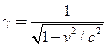Chapter 33, Problem 48PE

Chapter
Section
Textbook Problem

Integrated ConceptsPlans for an accelerator that produces a secondary beam of K−mesons to scatter from nuclei, for the purpose of studying the strong force, call for them to have a kinetic energy of 500 MeV. (a) What would the relativistic quantitybe for these particles? (b) How long would their average lifetime be in the laboratory? (c) How far could they travel in this time?

To determine

(a)

The relativistic quantity for 500 MeV K mesons.

Explanation

Given:

Kinetic energy of K mesons =500 MeV

Formula used:

Total energy of any relativistic particle, E=γE0=KE+E0

Where, γ relativistic quantity

E0 rest energy of the particle

KE kinetic energy of the particle

Calculation:

Since total energy, E=γE0=KE+E0 then

To determine

(b)

The average life time of K mesons in the laboratory.

To determine

(c)

The distance travelled by K mesons in the laboratory.

Still sussing out bartleby?

Check out a sample textbook solution.

See a sample solution

The Solution to Your Study Problems

Bartleby provides explanations to thousands of textbook problems written by our experts, many with advanced degrees!

Get Started